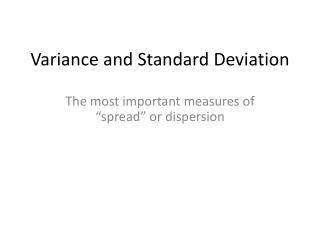Download PresentationVariance and Standard Deviation

# Variance and Standard Deviation

Download Presentation## Variance and Standard Deviation

- - - - - - - - - - - - - - - - - - - - - - - - - - - E N D - - - - - - - - - - - - - - - - - - - - - - - - - - -
##### Presentation Transcript

1. Variance and Standard Deviation The most important measures of “spread” or dispersion

2. Sample Variance The variance is the average squared deviation away from the mean. For a sample of size n, divide by n – 1. For a population, divide by n. Sample mean x

3. The standard deviation The standard deviation is simply the square root of the variance. The variance must always be calculated before the standard deviation.

4. Population mean μ σ and σ2 are pronounced “sigma” and “sigma squared”, respectively.

5. The coefficient of variation (CV) The coefficient of variation standardizes the standard deviation by dividing by the mean.

6. The Empirical Rule * This is actually a normal, continuous distribution, also known as a “bell curve”.

7. mode = median = mean mode < median < mean mode > median > mean

8. The amount of skewness is measured using Pearson’s coefficient of skewness (sk):